Computers, Materials & ContinuaDOI:10.32604/cmc.2022.021015Article

Artificial Intelligence Based Solar Radiation Predictive Model Using Weather Forecasts

1Department of Electrical and Electronics Engineering, University College of Engineering, Panruti, 607106, India
2Department of Mechanical Engineering, Rohini College of Engineering & Technology, Palkulam, 629401, India
3Department of Electrical and Electronics Engineering, Vignan's Institute of Information Technology, Andra Pradesh, 530046, India
4Department of Electrical and Electronics Engineering, University College of Engineering, BIT Campus, Tiruchirappalli, 620024, India
5Department of Computer Science, College of Computer and Information Sciences, Princess Nourah Bint Abdulrahman University, Saudi Arabia
6Department of Computer Science, King Khalid University, Muhayel Aseer, Saudi Arabia & Faculty of Computer and IT, Sana'a University, Sana'a, Yemen
7Department of Natural and Applied Sciences, College of Community-Aflaj, Prince Sattam bin Abdulaziz University, Saudi Arabia
*Corresponding Author: Sathish Babu Pandu. Email: psathishbabume@gmail.com
Received: 19 June 2021; Accepted: 27 July 2021

Abstract: Solar energy has gained attention in the past two decades, since it is an effective renewable energy source that causes no harm to the environment. Solar Irradiation Prediction (SIP) is essential to plan, schedule, and manage photovoltaic power plants and grid-based power generation systems. Numerous models have been proposed for SIP in the literature while such studies demand huge volumes of weather data about the target location for a lengthy period of time. In this scenario, commonly available Artificial Intelligence (AI) technique can be trained over past values of irradiance as well as weather-related parameters such as temperature, humidity, wind speed, pressure, and precipitation. Therefore, in current study, the authors aimed at developing a solar irradiance prediction model by integrating big data analytics with AI models (BDAAI- SIP) using weather forecasting data. In order to perform long-term collection of weather data, Hadoop MapReduce tool is employed. The proposed solar irradiance prediction model operates on different stages. Primarily, data preprocessing take place using various sub processes such as data conversion, missing value replacement, and data normalization. Besides, Elman Neural Network (ENN), a type of feedforward neural network is also applied for predictive analysis. It is divided into input layer, hidden layer, load-bearing layer, and output layer. To overcome the insufficiency of ENN in choosing the value of weights and hidden layer neuron count, Mayfly Optimization (MFO) algorithm is applied. In order to validate the performance of the proposed model, a series of experiments was conducted. The experimental values infer that the proposed model outperformed other methods used for comparison.

Keywords: Solar irradiation prediction; weather forecast; artificial intelligence; Elman neural network; mayfly optimization

1  Introduction

In general, sixty percent of a building's energy is consumed for ventilation, air-conditioning, and heating functions [1,2]. This energy could be saved through optimal control of heating and air-conditioning operations of the building. One of the major problems faced in terms of future global energy source is the combination of renewable energy source (mainly non-predictable ones such as solar and wind) to produce energy from current or upcoming energy sources. It is a must for an electrical operator to guarantee a proper balance between electricity production and consumption at any time. However, the operator faces several challenges during most of the times to preserve this balance with controllable and conventional energy production methods, mostly in small or not interrelated (i.e., isolated) electricity networks (that originate in an island, for example). The consistency of electric grid is decided based on the capacity of network to meet the unexpected and expected variations (i.e., in terms of consumption and production) and conflicts, while at the same time, preserving continuity and quality of facility to the consumers in a seamless manner. Afterwards, the energy provider should be able to handle the network with several time-based horizons .

A combination of renewable sources, connected with an electric system, complicates the network management process and consistency of consumption or production balance, owing to its unpredictable and intermittent environment . Solar energy production is a non-controllable and intermittent energy source due to which various challenges are faced like local power quality, stability issues, and voltage fluctuations. Therefore, predicting the output power of solar PV system is essential for efficient functioning of electrical network or optimum management of energy flows that occur in solar PV system . It is also required in electric network scheduling, resource estimation, optimum management of storage with stochastic production, congestion management, cost reduction in the production of electricity, and finally trade the energy generated in electricity market. It has become highly significant to predict the production of energy from solar PV since there is a significant rise in solar power production in the recent years. To prevent huge differences in renewable electricity generation, it is essential to involve the whole predictive system operation with storage results.

1.1 Role of Artificial Intelligence in Solar Irradiation Prediction

Artificial Intelligence (AI) technique has been applied in the recent years to predict performance improvement in SIP with regards to its capacity for simulating nonlinear and complex relations and manage the lost information [6,7]. Various AI methods have been proposed earlier to predict SR methods like data mining, Fuzzy Logic (FL), support vector regression, genetic programming, regression tree, and Artificial Neural Network (ANN). Amongst the AI methods proposed so far, Adaptive Neuro-Fuzzy Inference System (ANFIS), a combination of ANN and FL techniques, is considered as one of the most effective models. Numerous investigations have demonstrated that ANFIS method is highly effective in predicting SR . For instance, hybrid and classical ANFIS methods are combined with ANFIS through Differential Evolution Algorithm (DEA), Particle Swarm Optimization (PSO), and Genetic Algorithm (GA) techniques. While these algorithms have been utilized in the prediction of monthly global SR from distinct metrological variables such as minimum and maximum rainfall, air temperature, sunshine time and clearness index, when positioned in Kuala Terengganu, Malaysia. The outcomes exhibited that a hybrid ANFIS-PSA achieved optimum SR prediction in comparison with other techniques.

Traditional methods like Multiple Linear Regression (MLR) and distinct kinds of AI techniques involving ANFIS have been established earlier to predict everyday global SR in Iraq by distinct metrological variables. The outcomes demonstrated that ANFIS offers precise outcomes than other prediction methods. A relative study conducted upon distinct AI methods in predictive SR exposed that ANFIS is one of the most appropriate methods for simulating SR. This is attributed to its capability to conquer the uncertainties related to time-sequential data. But, the main challenge of this method i.e., ANFIS is the change in hyper variables such as optimization of membership variable functions. Consequently, the research works conducted earlier combined classical ANFIS method with several optimization techniques to improve its efficiency. However, the efficiency of the present hybrid ANFIS method is too inspiring. However, its predictive ability needs improvement by assuming the significance of SR accuracy measurement. Moreover, one of the main drawbacks of present SR predictive method is its demand for several parameters as input. These parameters could not be made easily available due to lack of monitoring network.

1.2 Paper Contributions

The current study introduces an effective solar irradiance prediction model by integrating big data analytics and AI models (BDAAI- SIP) and weather forecast data is applied in this model. To manage the long-term collection of weather data, Hadoop MapReduce tool is utilized. At the beginning, the presented BDAAI-SIP model undergoes data preprocessing to boost the quality of weather-related data. Besides, Elman Neural Network (ENN), a type of Feedforward Neural Network (FFNN) is applied for predictive analysis. It can be separated as input layer, hidden layer, load-bearing layer, and output layer. To optimize the parameters, Mayfly Optimization (MFO) algorithm is used. In order to validate the efficacy of the proposed BDAAI-SIP model, a set of simulations was conducted. In short, the contributions of the paper are summarized herewith.

•   A novel BDAAI-SIP model is proposed to predict solar irradiation with the help of weather forecasting data.

•   AI-based preprocessing is performed through three different ways such as data conversion, missing value replacement, and data normalization.

•   ENN model, comprising of load-bearing layer, is employed for prediction purposes.

•   In order to tune the weights and hidden layer neuron count in ENN model, MFO algorithm is applied.

•   Parameter optimization of ENN model further helps in increasing the predictive results of the proposed BDAAI-SIP model

•   The performance of BDAAI-SIP model was validated under several aspects and a comparative analysis was made.

1.3 Paper Organization

Rest of the sections in this paper are organized as given herewith. Section 2 offers the existing works related to SIP. Section 3 introduces the system methodology of the proposed BDAAI-SIP model. Section 4 validates the performance of BDAAI-SIP model and Section 5 concludes the paper.

2  Prior Works on Solar Irradiation Prediction Models

Several investigations have been conducted earlier with regards to Model Predictive Control (MPC), an optimal control approach that is introduced to assure effective system operation and control the air-conditioning process. Numerous researches have established the influence of decreasing the energy consumption of a building via MPC. The efficiency of MPC control is influenced by accurate information about hourly load prediction of a building. While this load consumption requirement gets influenced by climate data of the upcoming day. Thus, most of the methods require weather forecasting data. The general aspects that influence the loads are solar irradiance and outside air temperature. Though it is easy to predict outside air temperature due to small hourly variations, it is challenging to predict the real hourly values of solar irradiance.

In prior MPC investigations, solar irradiance prediction technique has been rarely stated. Several investigations in the literature utilized the information offered by energy analyses program. Otherwise, the studies considered the complete forecasted information about the quantity of solar irradiance in solar irradiance predictive method . In general, solar irradiance predictive technique is either based on data or physics . Physical method is commonly established depending upon solar geometry to create an experimental connection between solar irradiance data and meteorological variables measured in previous monitoring areas . Black  established a method to predict solar irradiance by examining the connection between sky cover and solar irradiance data collected for a period of 3 years in an area. Likewise, Samimi  established a solar irradiance method with high accuracy in which the researcher utilized climate data of Iran collected over 17 years.

Paltridge et al.  proposed a physics-based solar irradiance prediction method utilizing several climate variables like long-term accumulated data, humidity, wind, and precipitation. However, according to Premalatha et al. , to define solar irradiance coefficient in an area under study, physics-based weather prediction method needs long-term measured data or information that is complex for protecting, in typical weather predicting data. Thus, this method could not be employed in the prediction of upcoming day solar irradiance. Solar irradiance method that depends upon physical method, is established to calculate annual/monthly overall solar irradiance, instead of real-world predictive methods like MPC application .

Lago et al.  stated that the NN framework is beneficial in prediction and evaluation of the time sequential information with high arbitrariness. Jiang  stated that ANN predictive method demonstrates high accuracy than the experimental physical solar irradiance predictive method. Solar irradiance predictive method was introduced earlier based on learning data and it integrates several learning techniques based on the objective. Sharma et al.  proposed a method that grasps the scenario in 15 min period whereas, Kemoku et al.  conducted an investigation using FFNN to predict upcoming day solar irradiance by learning solar irradiance information for previous 6 years in Japan. Ahmad et al.  performed an investigation to identify the optimal integration of input variables to predict the solar irradiance including climate integration of 12 conditions of solar irradiance predictive in New Zealand.

Benmouiza et al.  presented a learning technique different from existing ANN models to predict solar irradiance. The investigations on ANN-based solar irradiance predictive method are widely performed and in recent times, the predictive method does not utilize the information attained from developed land. In order to predict the local solar irradiance, Rodríguez et al.  proposed an ANN method that grasps information attained from a satellite. This study considered six years data collected from various places. Srivastava et al.  introduced a solar irradiance predictive method which analyzes a major quantity of satellite data collected from different European countries. This method was utilized for studying nine years of climate conditions in 21 cities across Europe and US.

3  The Proposed BDAAI- SIP Model

The overall system architecture is shown in Fig. 1. As shown in the figure, the proposed BDAAI-SIP model undergoes three major processes namely, data preprocessing, predictive analysis, and parameter optimization. Besides, the Hadoop MapReduce tool is also applied to handle the massive collection of weather forecast data.Figure 1: The overall working process of BDAAI-SIP model

3.1 Overall System Methodology

The processes involved in overall system methodology are briefed herewith.

•   Initially, weather-related data is fed as input to BDAAI-SIP model and it is analyzed in big data analytics environment.

•   Then, preprocessing is performed through three different stages such as data conversion, missing value replacement, and data normalization.

•   Followed by, ENN-based predictive model is applied for prediction. This model makes use of a load bearing layer that transmits the state information and memory.

•   Next, the parameter optimization of ENN model takes place using MFO algorithm to optimally determine the values of weights and hidden layer neuron count.

•   Lastly, the performance of the BDAAI-SIP model is validated on benchmark dataset and the results are investigated in terms of different aspects.

In order to manage big data, Hadoop ecosystem and its components are widely applied. In a distributed atmosphere, Hadoop is a type of open-source design that allows a stakeholder to process big data on computer clusters with the help of simple programming systems. Since a single server has thousands of nodes, it can be simulated to involve improved scalability as well as fault tolerance. The three major components of Hadoop are MapReduce, Hadoop Distributed File System (HDFS), and Hadoop YARN.

3.2.1 Hadoop Distributed File System (HDFS)

Google File System (GFS) demonstrates HDFS as a structure of variety with master/slave, where master has more than one data node and is named after actual data whereas different name nodes are known to be metadata.

In order to offer massive scalability on thousand Hadoop clusters, Hadoop Map Reduce is utilized in the name of Apache Hadoop heart, a programming structure. To process huge information on massive clusters, MapReduce is utilized. Two essential stages are involved in MapReduce job modeling namely, Reduce and Map stage. All the stages contain key value pairs from input as well as output i.e., from the file system, combined output as well as input of the job are stored. The framework handles different tasks such as task scheduling, re-execution of the failed tasks and controlling the tasks. MapReduce framework contains one slave node control and a single master resource manager in every cluster node.

Hadoop YARN is a method utilized to manage the clusters. Based on the experience obtained from initial Hadoop generation, the second Hadoop generation is processed as an essential feature. YARN functions as a central structure and resource manager over Hadoop clusters in order to deal security, reliable functions, and data governance tools. In big data management, another platform device and components are installed on Hadoop framework.

3.3 Data Preprocessing

Data pre-processing is an important part of AI technique and can considerably enhance the efficiency of the model. During data preprocessing in BDAAI model, the data initially undergoes conversion process in which the categorical values are transformed into numerical values. Besides, missing values’ replacement occurs to replace the missing values with alternate ones. Finally, min-max based data normalization process is applied to adjust the dataset to a uniform scale. In this technique, maximal and minimal values from a set data are examined. Every other data is normalized to these values. The purpose of normalization is to make the minimum value to zero and maximum value to one so that every other data is distributed in the range of 0 to 1. Eq. (1) provides the equation for min.-max. normalization.

MinMax.Norm=xxmin/xmaxxmin(1)

3.4 Elman Neural Network (ENN)-Based Predictive Model

ENN was presented by J. L. Elman to solve speech signal process in 1990 . ENN is a dynamic recurrent network. On the contrary to conventional BPNN, ENN has a specific layer called context layer that enables this network with the capability to learn the time changing patterns. Due to this feature, ENN is highly appropriate for separate time sequence problems. ENN framework is given in Fig. 2. Excluding the context layer, the remaining portions are assumed to be conventional multiple-layer networks. The context layer shown in Fig. 2 is acquired from the outputs of hidden layer. Later, the outcomes of context layer are fed as input to the hidden layer along with the following set of external input layer data. The data, collected from earlier times, is reused and stored in these features.Figure 2: Structure of ENN model

ENN is shown in Fig. 2 has a n dimension external input layer which is denoted by x1(t)=[x1,1(t), x1,2(t), . . . , x1,n(t)]T, now t represents tth input series. For ease, the output of previous layer is implemented in n neuron, and the output vector of the layer is given by y(t)=[y1(t), y2(t), . . . , yn(t)]T. The neuron present between context and hidden layers are individually equivalent. Later, the count of neuron context layer is denoted by m, that is similar to hidden layer. The hidden layer input from the context layer is determined by x2(t)=c(t1)=[c1(t1),c2(t1),,cm(t1)]T. The whole input vector of the network is given by

x(t)=[x1T(t)x2T(t)]T=[x1,1(t),x1,2(t),,x1,n(t),c1(t1),,cm(t1)]T=[x1(t),x2(t),,xk(t)]T,(2)

where k=m+n. Matrixes among three layers are denoted by Whi(t), Whc(t) and Woh(t) correspondingly. It is necessary to recognize the size of these matrices . With the evaluation of dimension of every layer, Whi(t)Rm×n, Whc(t)Rm×m and Woh(t)Rn×m are attained.

Here, y(t) denotes the original output of this network and d(t) represents the desired output vector. When the activation function is selected as sigmoid function, then y(t) is calculated as follows

yi(t)=f(vio(t))=11+exp(vio(t)),i=1,2,,n,(3)

vio(t)=j=1mWjioh(t)×hj(t),i=1,2,,n.(4)

The input of the hidden layer is comprised of two portions namely context and external inputs, so, Wh(t)=[Whi(t) Whc(t)]Rm×k. With whole input vector x(t) and sigmoid activation function, the output of hidden layer is given by

hj(t)=f(vjh(t))=11+exp(vjh(t)),j=1,2,,m,(5)

vjh(t)=l=1kWjlh(t)×xl(t),j=1,2,,m(6)

The aim of this network is to reduce the error:

E(t)=||e(t)||22,(7)

e(t)=d(t)y(t)(8)

To reduce(t), the update of every weight matrix is calculated by the formula given below.

Woh(t+1)=Woh(t)μE(t)Woh(t)=Woh(t)+μy(t)e(t)hT(t)(9)

Wh(t+1)=Wh(t)μE(t)Wh(t)=Wh(t)+μh(t)[Woh(t)]Ty(t)e(t)xT(t)(10)

here, μ represents the learning rate, and

y(t)=diag[f(v1o(t))f(v2o(t))f(vno(t))]Rn×n(11)

h(t)=diag[f(v1h(t))f(v2h(t))f(vmh(t))]Rm×m(12)

3.5 Mayfly Optimization (MFO) Algorithm Based Parameter Optimization

The choice of parameters in ENN model is a crucial element to attain an effective classification outcome. Most of the ML models include multiple parameters that need to be optimized. Since trial-and-error method is infeasible, metaheuristic optimization based-MFO algorithm is applied in the selection of parameters. In general, the predictive error function acts as the objective function of MFO algorithm . Among mayflies, the swarms for MO technique are divided into male as well as female separately. When male mayflies are stronger, it subsequently acts as the optimal factor in optimization. When separate optimization is compared with that of swarms in PSO technique, the individuals in MO technique upgrade their location based on its present location, pi(t) and velocity vi(t) at present iteration:

pi(t+1)=pi(t)+vi(t+1)(13)

Every male mayfly and female mayfly upgrades its location in Eq. (13). But, its velocity gets upgraded in different ways.

3.5.1 Movements of Male Mayflies

Male mayfly swarms are performed with exploration or exploitation process during iterations. The velocity gets upgraded based on its present fitness value, f(xi) and historical optimal fitness value in paths f(xhi). When f(xi)>f(xhi), the male mayflies upgrades their velocities based on its current velocities. This value is combined with the distance between them and the global optimal location. The historical optimal path is defined herewith.

vi(t+1)=gvi(t)+a1eβrp2[xhixi(t)]+a2eβrq2,[xgxi(t)](14)

where g implies the variable that gets reduced linearly from maximal value to lesser one. a1, a2, andβ are three constants to balance the values. rp and rg are two variables that are generally utilized in informing the Cartesian distance amongst the individuals and their historical optimal position with that of the global optimal location in swarms. Cartesian distance is the second norm to distance array and is given below.

||xixj||=k=1n(xikxjk)2(15)

Conversely, when f(xi)<f(xhi), the male mayflies upgrades their velocities in the present one with an arbitrary dance coefficient d:

vi(t+1)=gvi(t)+dr1(16)

where, r1 represents the arbitrary number from uniform distribution and is chosen in the domain ranged between −1 and 1.

3.5.2 Movements of Female Mayflies

Female mayflies upgrade their velocities through various styles. Biologically speaking, female winged-mayflies live only for a time span of 1–7 days. Thus, the female mayflies rush to detect the male mayflies for mating and reproduction. So, the velocities of female mayflies are upgraded according to male mayflies since it is required for mating purpose. In this MO technique, top optimal female and male mayflies are defined as the initial mate, and the second optimal female, male mayflies are defined as second mates, etc. Therefore, the i-th female mayfly, when f(yi)<f(xi), is denoted by

vi(t+1)=gvi(t)+a3eβrmf2[xi(t)yi(t)](17)

where, a3 signifies another constant and is utilized for balancing the velocities. rm implies the Cartesian distance between them. In contrast, when (yi)<f(xi), the female mayflies upgrade its velocities in the present one with other arbitrary dance, fl:

vi(t)=gvi(t)+flr2(18)

where, r2 denotes the arbitrary number from a uniform distribution in the domains ranged between −1 and 1.

3.5.3 Mating of Mayflies

Every top half female and male mayfly is mated and produce a pair of children. Its offspring are arbitrarily developed by their parents:

offspring1=Lmale+(1L)femaleoffspring2=Lfemale+(1L)male(20)

where L represents the arbitrary numbers from Gaussian distribution.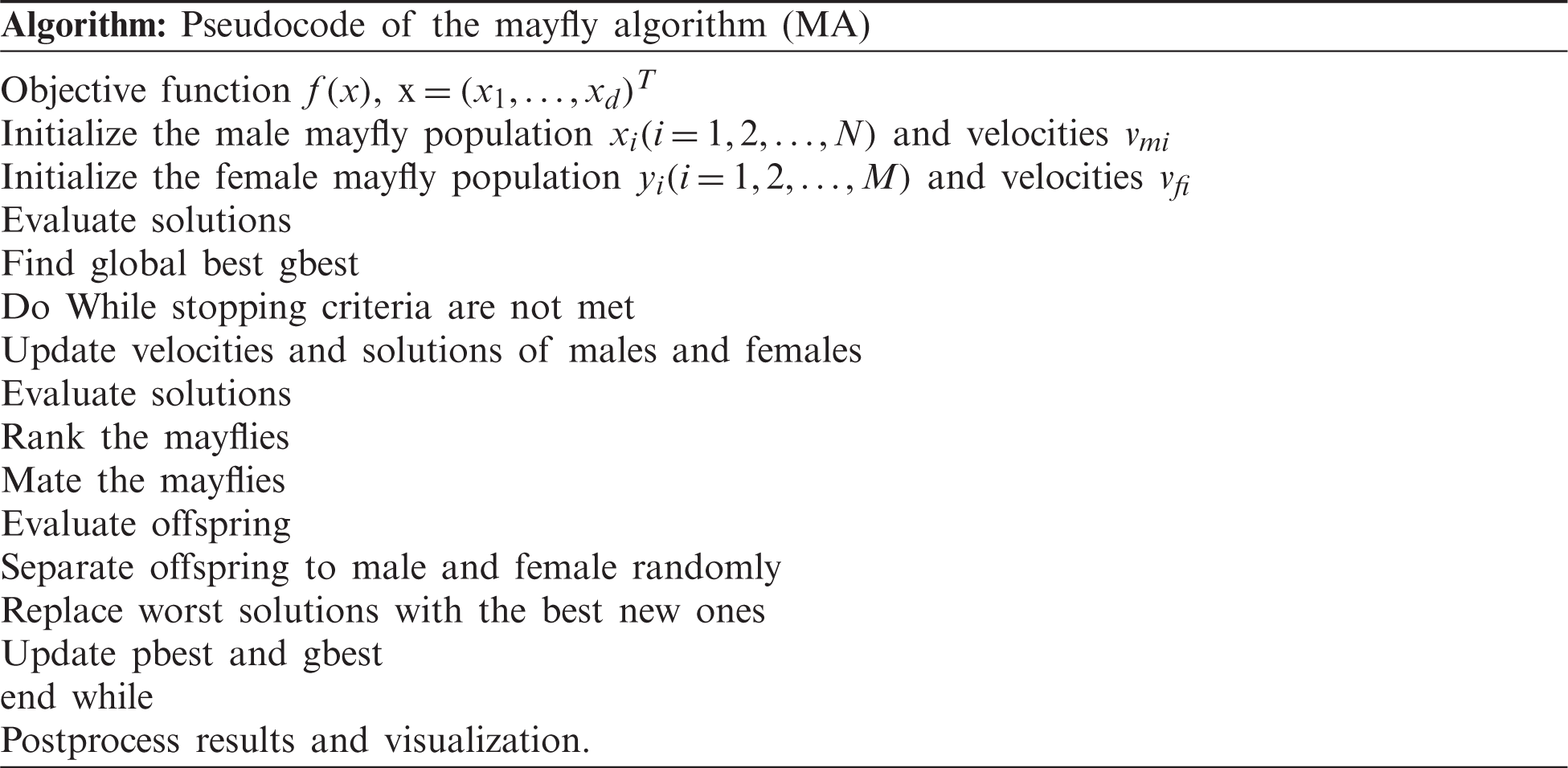4  Performance Validation

In order to assess the predictive performance of BDAAI-SIP model, a set of simulations was conducted using HI-SEAS Solar Irradiance Prediction dataset sourced from Kaggle repository . The dataset holds weather-related details from HI-SEAS Habitat in Hawaii. Particularly, the dataset comprises of the following parameters namely, Solar Irradiance (W/m2), Temperature (°F), Barometric Pressure (Hg), Humidity (%), Wind Direction (°), Wind Speed (mph), and Sun Rise/Set Time. The results were validated under two measures such as Mean Square Error (MSE) and Root Mean Square Error (RMSE) methods.

Tab. 1 and Fig. 3 shows the results of the analysis achieved by BDAAI-SIP model in terms of MSE and RMSE on the applied dataset. From the table, it is evident that the BDAAI-SIP model attained minimal MSE and RMSE values for training, testing, and validation datasets. For instance, on the applied fold-1, the BDAAI-SIP model achieved the least MSE values such as 8596.998, 8596.998, and 8951.052 on the applied training, testing, and validation datasets respectively. Similarly, the BDAAI-SIP model obtained minimal RMSE values such as 92.72, 93.68, and 94.61 on the applied training, testing, and validation datasets respectively. Likewise, on the applied fold-3, the BDAAI-SIP technique accomplished minimum MSE values such as 8794.688, 8998.420, and 8777.816 on the applied training, testing, and validation datasets correspondingly.

Concurrently, the BDAAI- SIP approach obtained lesser RMSE values such as 93.78, 94.86, and 93.69 on the applied training, testing, and validation datasets respectively. At the same time, on the applied fold-5, the BDAAI-SIP model reached the least MSE values such as 8253.723, 8675.060, and 8738.510 on the applied training, testing, and validation datasets respectively. Simultaneously, the BDAAI-SIP method accomplished low RMSE values such as 90.85, 93.14, and 93.48 on the applied training, testing, and validation datasets correspondingly. In addition, on the applied fold-7, the BDAAI- SIP method yielded minimum MSE values such as 8326.563, 8535.912, and 8764.704 on the applied training, testing, and validation datasets correspondingly. Followed by, the BDAAI-SIP model obtained lesser RMSE values such as 91.25, 92.39, and 93.62 on the applied training, testing, and validation datasets respectively. Moreover, on the applied fold-10, the BDAAI-SIP technique obtained the least MSE values such as 8753.474, 8222.862, and 8796.564 on the applied training, testing, and validation datasets respectively. At last, the BDAAI-SIP approach attained lesser RMSE values such as 93.56, 90.68, and 93.79 on the applied training, testing, and validation datasets correspondingly.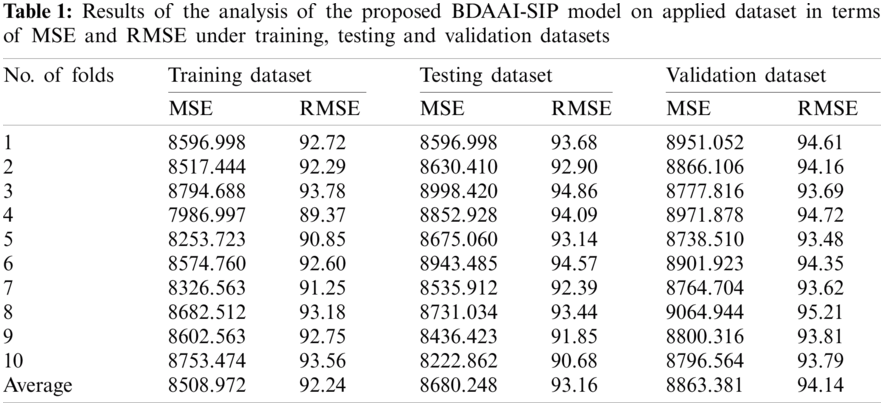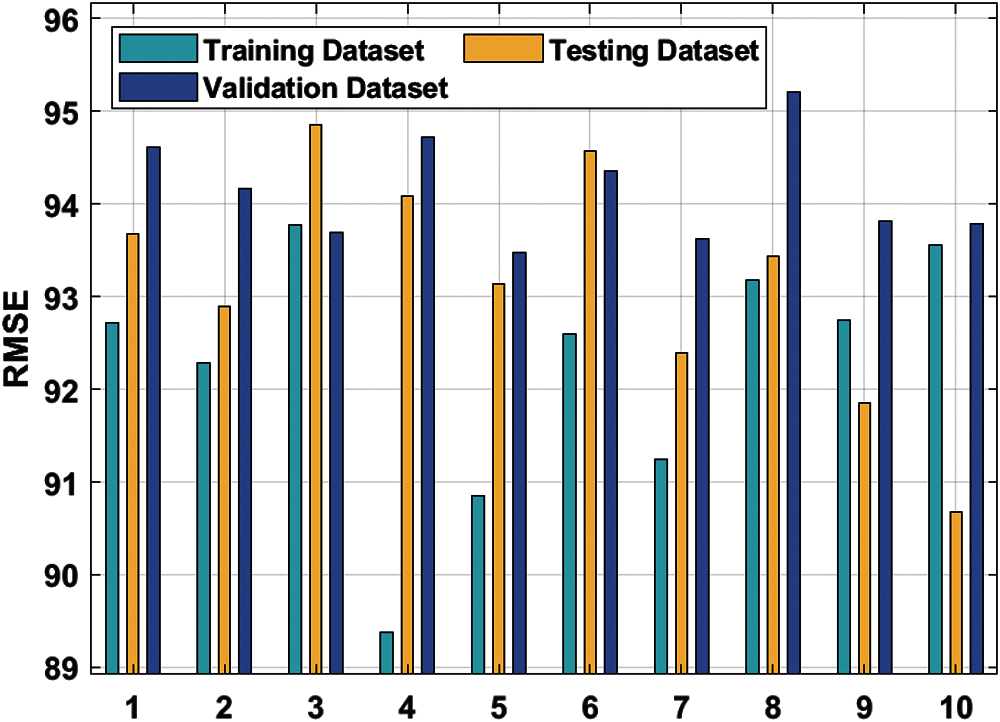Figure 3: RMSE analysis of BDAAI-SIP model

Fig. 4 shows the results of average RMSE analysis for the proposed BDAAI-SIP model on the applied dataset. From the figure, it is understood that the proposed BDAAI-SIP model has demonstrated better results since it achieved an effective performance through maximum average RMSE values such as 92.24, 93.16, and 94.14 on the applied training, testing, and validation datasets respectively.

Tab. 2 and Fig. 5 shows the results yielded by BDAAI-SIP model under varying times in terms of predicted irradiance. From the table, it can be understood that BDAAI-SIP model appropriately predicted the irradiance. In other terms, the difference from actual true value is considerably lower than the compared methods. For instance, for a time period of 8 h with true value being 23.380 W/m2, the BDAAI-SIP model has predicted the irradiance to be 42.380, whereas other methods such as LSTM, BPNN, Persistence, and LR models predicted inferior results for predictive irradiance such as 058.640, 148.145, 080.338, and 112.886 W/m2 respectively.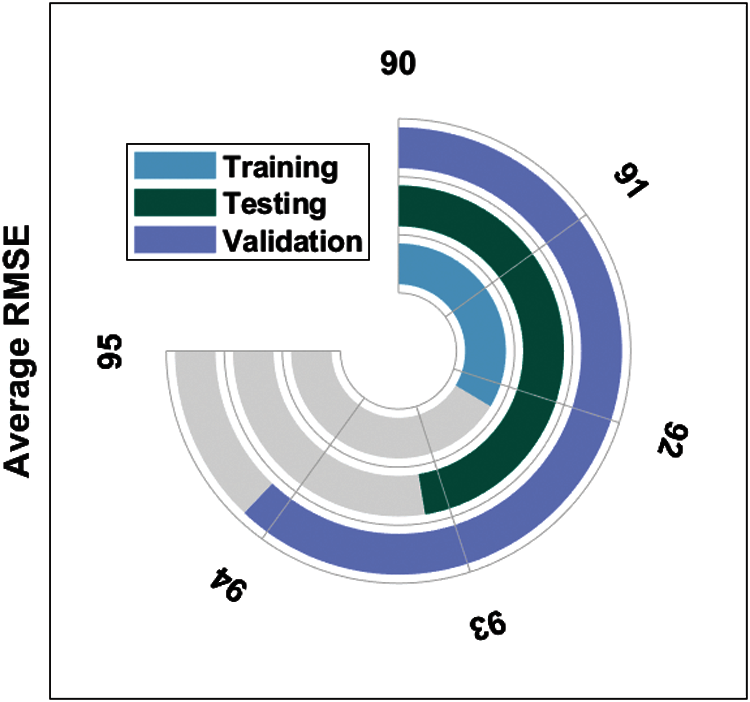Figure 4: Average RMSE analysis of BDAAI-SIP model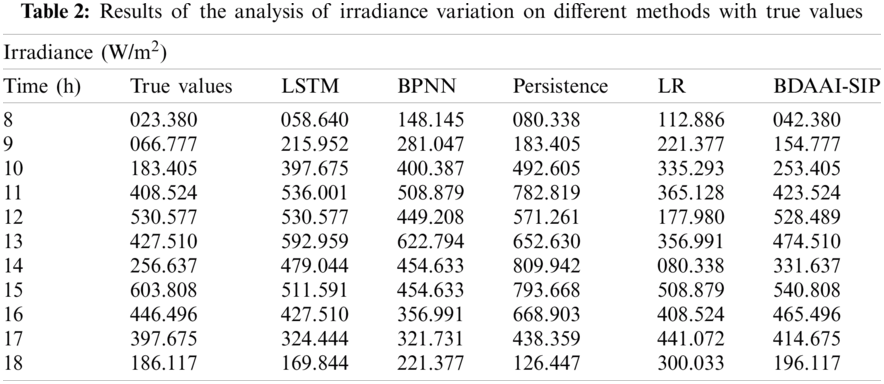Besides, for a time period of 10 h, with its true value being 183.405 W/m2, the BDAAI-SIP model predicted the irradiance to be 253.405. While other methods such as LSTM, BPNN, Persistence, and LR approaches showcased inferior results with predictive irradiance values being 397.675, 400.387, 492.605, and 335.293 W/m2 respectively. Eventually, for a time period of 12 h, with a true value of 530.577 W/m2, the BDAAI-SIP model predicted the irradiance to be 528.489, whereas other methods such as LSTM, BPNN, Persistence, and LR approaches produced inferior outcomes with predictive irradiance values being 530.577, 449.208, 571.261, and 177.980 W/m2 respectively. Meanwhile, for a time period of 14 h, with a true value of 256.637 W/m2, the BDAAI-SIP model predicted the irradiance to be 331.637, whereas other methods such as LSTM, BPNN, Persistence, and LR techniques portrayed inferior results with the predictive irradiance of 479.044, 454.633, 809.942, and 080.338 W/m2 correspondingly.Figure 5: Results of the analysis of BDAAI-SIP model under distinct irradiance

Likewise, for a time period of 16 h, with 446.496 W/m2 true value, the BDAAI- SIP technique predicted the irradiance to be 465.496. On the other hand, other methods such as LSTM, BPNN, Persistence, and LR models exhibited inferior results with predictive irradiance values being 427.510, 356.991, 668.903, and 408.524 W/m2 respectively. At last, for a time period of 18 h, with a true value of 186.117 W/m2, the BDAAI- SIP model predicted the irradiance to be 196.117, whereas other models namely, LSTM, BPNN, Persistence, and LR models yielded inferior outcomes with predictive irradiance values being 169.884, 221.337, 126.447, and 300.033 W/m2 correspondingly.

Tab. 3 and Fig. 6 provides a brief comparison of MSE and RMSE values obtained by the proposed BDAAI-SIP model and existing methods on the applied training and testing datasets [29,30]. From the results, it is evident that the LR model showcased ineffective predictive results with its RMSE values being 200.991 and 195.875 W/m2 on training and testing datasets respectively. At the same time, the Persistence model displayed a slightly higher outcome with its RMSE value being 177.031 W/m2 on the applied testing dataset. Next to that, the BPNN model has yielded moderate RMSE values such as 17726.47 and 22585.43 W/m2 on training and testing datasets respectively. The RNN model accomplished an improved outcome with its RMSE values being 15055.29 and 122.700 W/m2 on training and testing datasets respectively. In line with this, both LSTM and GRU methods demonstrated a closer and reasonable outcome over other methods. But the presented model outperformed other models through better results i.e., least RMSE values such as 092.240 and 93.160 W/m2 upon training and testing datasets respectively. From the above discussed results of the analysis, it is evident that the presented BDAAI-SIP model is an effective predictive tool for solar irradiation.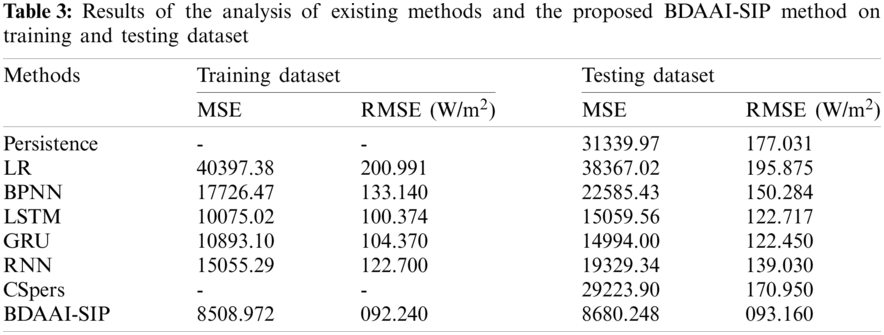Figure 6: Comparative analysis of BDAAI-SIP model with existing techniques

5  Conclusion

The current research article designed a novel BDAA-SIP model to predict the solar irradiance using weather forecast data. Initially, weather related data are fed as input to BDAAI-SIP model which undergoes analysis on big data environment. Then, preprocessing is conducted through three different stages such as data conversion, missing value replacement, and data normalization. Followed by, ENN-based predictive model is applied in the prediction. This model makes use of a load bearing layer that transmits state information and memory. Next, the parameter optimization of ENN model takes place through MFO algorithm in order to optimally determine the values of weights and count of hidden layer neurons. Lastly, the performance of the proposed BDAAI-SIP model was validated on benchmark datasets and the results were investigated under different aspects. To examine the efficacy of BDAAI- SIP model, a set of simulations was conducted. The experimental values highlight that the proposed method yielded better performance than the compared methods. As a part of future scope, the presented model can be extended to design the next day SIP model using weather forecast data.

Funding Statement: The authors extend their appreciation to the Deanship of Scientific Research at King Khalid University for funding this work under Grant Number (RGP1/147/42), Received by Fahd N. Al-Wesabi. https://www.kku.edu.sa. This research was funded by the Deanship of Scientific Research at Princess Nourah bint Abdulrahman University through the Fast-track Research Funding Program.

Conflicts of Interest: The authors declare that they have no conflicts of interest to report regarding the present study.

References

1.  B. Jeon and E. J. Kim, “Next-day prediction of hourly solar irradiance using local weather forecasts and LSTM trained with non-local data,” Energies, vol. 13, no. 20, pp. 5258, 2020.

2.  A. Bolzoni, A. Parisio, R. Todd and A. Forsyth, “Model predictive control for optimizing the flexibility of sustainable energy assets: An experimental case study,” International Journal of Electrical Power & Energy Systems, vol. 129, pp. 106822, 2021.

3.  J. Ngarambe, G. Y. Yun and M. Santamouris, “The use of artificial intelligence (AI) methods in the prediction of thermal comfort in buildings: Energy implications of AI-based thermal comfort controls,” Energy and Buildings, vol. 211, pp. 109807, 2020.

4.  T. Ahmad, H. Zhang and B. Yan, “A review on renewable energy and electricity requirement forecasting models for smart grid and buildings,” Sustainable Cities and Society, vol. 55, pp. 102052, 2020.

5.  G. V. B. Kumar and K. Palanisamy, “A review of energy storage participation for ancillary services in a microgrid environment,” Inventions, vol. 5, no. 4, pp. 63, 2020.

6.  J. Uthayakumar, N. Metawa, K. Shankar and S. K. Lakshmanaprabu, “Intelligent hybrid model for financial crisis prediction using machine learning techniques,” Information Systems and e-Business Management, vol. 18, no. 4, pp. 617–645, 2020.

7.  H. Tao, A. A. Ewees, A. O. Al-Sulttani, U. Beyaztas, M. M. Hameed et al., “Global solar radiation prediction over north dakota using air temperature: Development of novel hybrid intelligence model,” Energy Reports, vol. 7, pp. 136–157, 2021.

8.  S. K. Lakshmanaprabu, K. Shankar, S. S. Rani, E. Abdulhay, N. Arunkumar et al., “An effect of big data technology with ant colony optimization based routing in vehicular ad hoc networks: Towards smart cities,” Journal of Cleaner Production, vol. 217, pp. 584–593, 2019.

9.  B. K. Jeon, E. J. Kim, Y. Shin and K. H. Lee, “Learning-based predictive building energy model using weather forecasts for optimal control of domestic energy systems,” Sustainability, vol. 11, no. 1, pp. 147, 2018.

10. S. K. Aggarwal and L. M. Saini, “Solar energy prediction using linear and non-linear regularization models: A study on AMS (American meteorological society) 2013–14 solar energy prediction contest,” Energy, vol. 78, pp. 247–256, 2014.

11. F. Wang, Z. Mi, S. Su and H. Zhao, “Short-term solar irradiance forecasting model based on artificial neural network using statistical feature parameters,” Energies, vol. 5, no. 5, pp. 1355–1370, 2012.

12. J. N. Black, “The distribution of solar radiation over the earth's surface,” Archiv Für Meteorologie, Geophysik und Bioklimatologie, Serie B, vol. 7, no. 2, pp. 165–189, 1956.

13. J. Samimi, “Estimation of height-dependent solar irradiation and application to the solar climate of Iran,” Solar Energy, vol. 52, no. 5, pp. 401–409, 1994.

14. G. W. Paltridge and D. Proctor, “Monthly mean solar radiation statistics for Australia,” Solar Energy, vol. 18, no. 3, pp. 235–243, 1976.

15. N. Premalatha and A. Valan Arasu, “Prediction of solar radiation for solar systems by using ANN models with different back propagation algorithms,” Journal of Applied Research and Technology, vol. 14, no. 3, pp. 206–214, 2016.

16. J. M. Vindel, J. Polo and L. F. Zarzalejo, “Modeling monthly mean variation of the solar global irradiation,” Journal of Atmospheric and Solar-Terrestrial Physics, vol. 122, pp. 108–118, 2015.

17. J. Lago, F. De Ridder and B. De Schutter, “Forecasting spot electricity prices: Deep learning approaches and empirical comparison of traditional algorithms,” Applied Energy, vol. 221, pp. 386–405, 2018.

18. Y. Jiang, “Computation of monthly mean daily global solar radiation in China using artificial neural networks and comparison with other empirical models,” Energy, vol. 34, no. 9, pp. 1276–1283, 2009.

19. V. Sharma, D. Yang, W. Walsh and T. Reindl, “Short term solar irradiance forecasting using a mixed wavelet neural network,” Renewable Energy, vol. 90, pp. 481–492, 2016.

20. Y. Kemmoku, S. Orita, S. Nakagawa and T. Sakakibara, “Daily insolation forecasting using a multi-stage neural network,” Solar Energy, vol. 66, no. 3, pp. 193–199, 1999.

21. A. Ahmad, T. N. Anderson and T. T. Lie, “Hourly global solar irradiation forecasting for New Zealand,” Solar Energy, vol. 122, pp. 1398–1408, 2015.

22. K. Benmouiza and A. Cheknane, “Forecasting hourly global solar radiation using hybrid k-means and nonlinear autoregressive neural network models,” Energy Conversion and Management, vol. 75, pp. 561–569, 2013.

23. A. L. Rodríguez, J. A. Ruiz-Arias, D. Pozo-Vázquez and J. T. Pescador, “Generation of synthetic daily global solar radiation data based on ERA-interim reanalysis and artificial neural networks,” Energy, vol. 36, no. 8, pp. 5356–5365, 2011.

24. S. Srivastava and S. Lessmann, “A comparative study of LSTM neural networks in forecasting day-ahead global horizontal irradiance with satellite data,” Solar Energy, vol. 162, pp. 232–247, 2018.

25. J. L. Elman, “Finding structure in time,” Cognitive Science, vol. 14, no. 2, pp. 179–211, 1990.

26. K. Xie, H. Yi, G. Hu, L. Li and Z. Fan, “Short-term power load forecasting based on elman neural network with particle swarm optimization,” Neurocomputing, vol. 416, pp. 136–142, 2020.

27. Z. M. Gao, J. Zhao, S. R. Li and Y. R. Hu, “The improved mayfly optimization algorithm with opposition based learning rules,” Journal of Physics: Conference Series, vol. 1693, pp. 12117, 2020.

28. Dataset, 2017. [Online]. Available: https://www.kaggle.com/callumdownie/hi-seas-solar-irradiance-prediction.

29. X. Qing and Y. Niu, “Hourly day-ahead solar irradiance prediction using weather forecasts by LSTM,” Energy, vol. 148, pp. 461–468, 2018.

30. B. Gao, X. Huang, J. Shi, Y. Tai and R. Xiao, “Predicting day-ahead solar irradiance through gated recurrent unit using weather forecasting data,” Journal of Renewable and Sustainable Energy, vol. 11, no. 4, pp. 43705, 2019.This work is licensed under a Creative Commons Attribution 4.0 International License, which permits unrestricted use, distribution, and reproduction in any medium, provided the original work is properly cited.